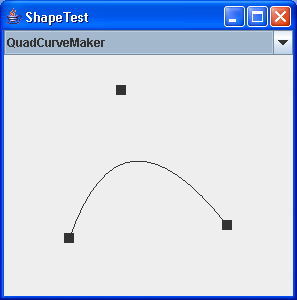# Union two rectangles : Shape « 2D Graphics GUI « Java

Union two rectangles

```
/*
* \$Id: RectUtils.java,v 1.2 2008/02/28 14:38:48 david Exp \$
*
* Copyright (c) 2008 Gaudenz Alder
*
*/

import java.awt.geom.Rectangle2D;

public class RectUtils {
/**
* Unions the pair of source <code>Rectangle2D</code> objects and puts the
* result into the returned <code>Rectangle2D</code> object. This method
* extends the Rectangle2D version by checking for null parameters, the
* returned value will also be <code>null</code> if the two input
* rectangles are <code>null</code>
*
* @param src1
*            the first of a pair of <code>Rectangle2D</code> objects to
*            be combined with each other
* @param src2
*            the second of a pair of <code>Rectangle2D</code> objects to
*            be combined with each other
*
*/
public static Rectangle2D union(Rectangle2D src1, Rectangle2D src2) {
Rectangle2D result = null;
if (src1 == null && src2 == null) {
result = null;
} else if (src1 != null && src2 != null) {
double x1 = Math.min(src1.getMinX(), src2.getMinX());
double y1 = Math.min(src1.getMinY(), src2.getMinY());
double x2 = Math.max(src1.getMaxX(), src2.getMaxX());
double y2 = Math.max(src1.getMaxY(), src2.getMaxY());
result = new Rectangle2D.Double();
result.setFrameFromDiagonal(x1, y1, x2, y2);
} else if (src1 != null) {
double x1 = src1.getMinX();
double y1 = src1.getMinY();
double x2 = src1.getMaxX();
double y2 = src1.getMaxY();
result = new Rectangle2D.Double();
result.setFrameFromDiagonal(x1, y1, x2, y2);
} else {
// only src2 is non-null
double x1 = src2.getMinX();
double y1 = src2.getMinY();
double x2 = src2.getMaxX();
double y2 = src2.getMaxY();
result = new Rectangle2D.Double();
result.setFrameFromDiagonal(x1, y1, x2, y2);
}
return result;
}
}

```

### Related examples in the same category

 1 Creating Basic Shapes 2 fillRect (int, int, int, int) method draws a solid rectangle 3 Creating a Shape Using Lines and Curves 4 Combining Shapes 5 Draw rectangles, use the drawRect() method. To fill rectangles, use the fillRect() method 6 Draw line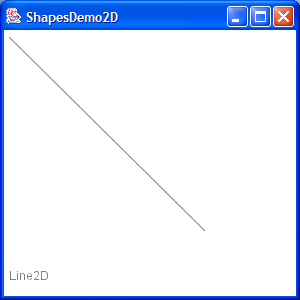7 Draw a Polygon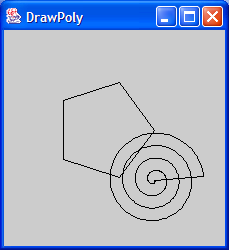8 Draw an oval outline 9 Draw a (Round)rectangle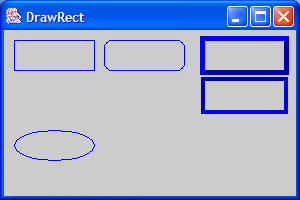10 Fill a polygon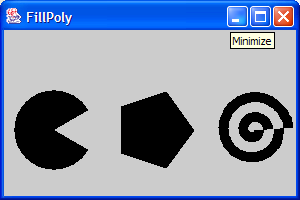11 Fill a solid oval 12 Fill a (Round)rectangle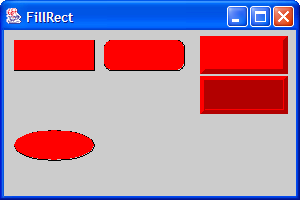13 Change font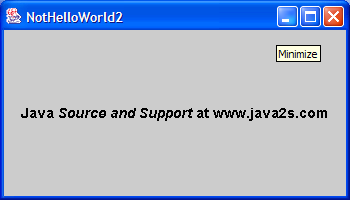14 Draw rectangle 2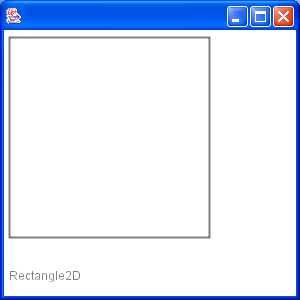15 Draw Arc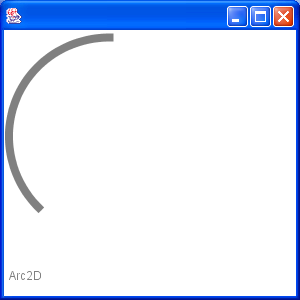16 Draw Ellipse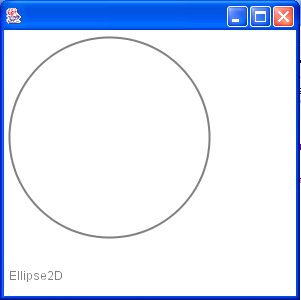17 Fill a Rectangle 2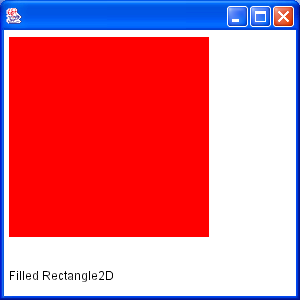18 Fill Arc 2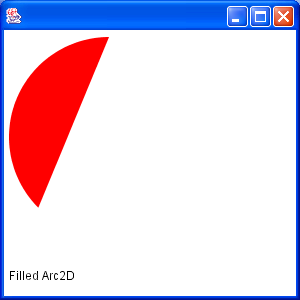19 Draw text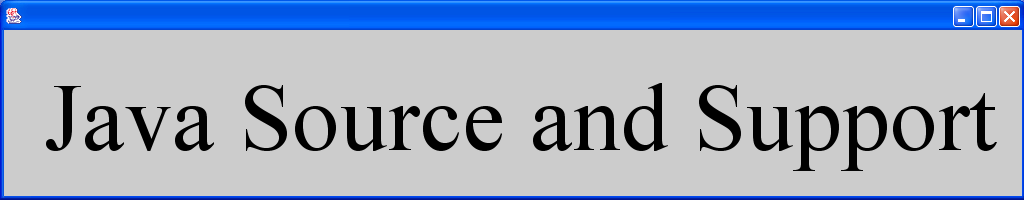20 Draw unicode string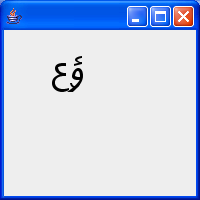21 Shape combine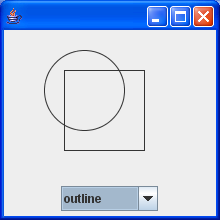22 Effects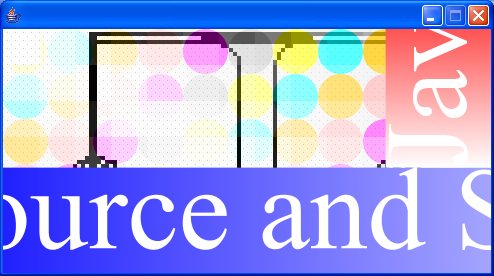23 Mouse drag and drop to draw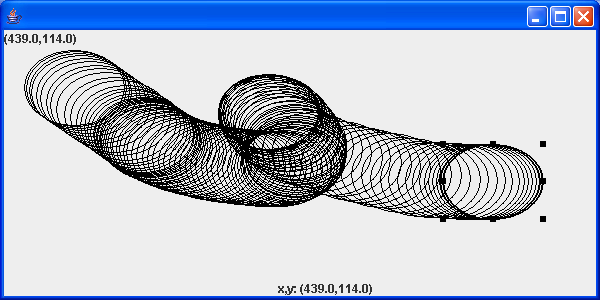24 Arc demonstration: scale, move, rotate, sheer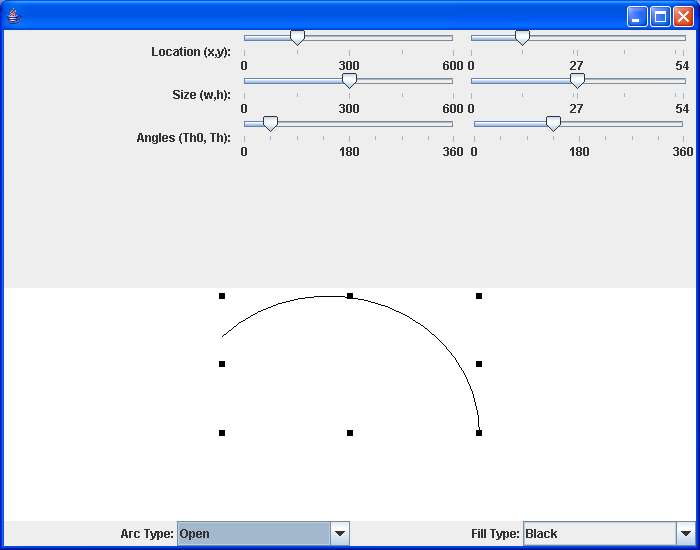25 Hypnosis Spiral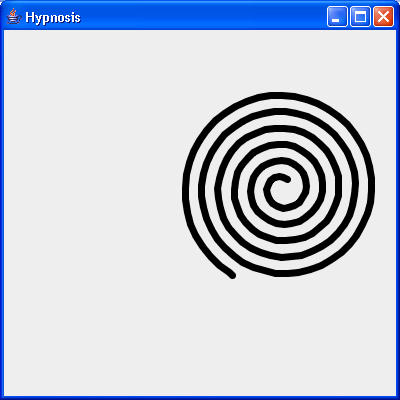26 GlyphVector.getNumGlyphs() 27 Resize a shape 28 Rectangle with rounded corners drawn using Java 2D Graphics API 29 Compares two ellipses and returns true if they are equal or both null. 30 Compares two lines are returns true if they are equal or both null. 31 Creates a diagonal cross shape. 32 Creates a diamond shape. 33 Creates a new Stroke-Object for the given type and with. 34 Creates a region surrounding a line segment by 'widening' the line segment. 35 Creates a triangle shape that points downwards. 36 Creates a triangle shape that points upwards. 37 Generate Polygon 38 Polygon with float coordinates. 39 Polyline 2D 40 Serialises a Shape object. 41 Tests two polygons for equality. If both are null this method returns true. 42 Calculate Intersection Clip 43 Draws a shape with the specified rotation about (x, y). 44 Checks, whether the given rectangle1 fully contains rectangle 2 (even if rectangle 2 has a height or width of zero!). 45 Reads a Point2D object that has been serialised by the writePoint2D(Point2D, ObjectOutputStream)} method. 46 Returns a point based on (x, y) but constrained to be within the bounds of a given rectangle. 47 RectListManager is a class to manage a list of rectangular regions. 48 Fill Rectangle2D.Double and Ellipse2D.Double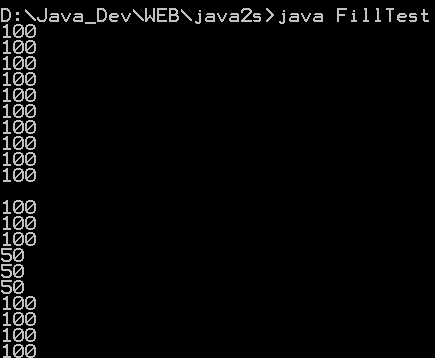49 This program demonstrates the various 2D shapes